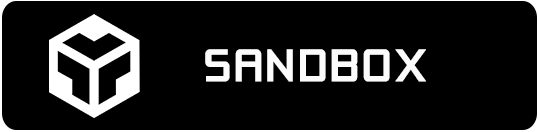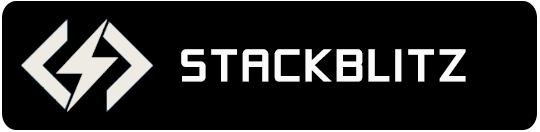# Angular Bullet Graph Overview

The Angular bullet graph component allows for a linear and concise view of measures compared against a scale.

The Ignite UI for Angular bullet graph component provides you with the ability to create attractive data presentations, replacing meters and gauges that are used on dashboards with simple yet straightforward and clear bar charts. A bullet graph is one of the most effective and efficient ways to present progress towards goals, good/better/best ranges, or compare multiple measurements in as little horizontal or vertical space as possible.

## Angular Bullet Graph Example

The following sample demonstrates how setting multiple properties on the same `IgxBulletGraphComponent` can transform it to completely different bullet graph.The bullet graph supports one scale, one set of tick marks and one set of labels. The bullet graph component also has built-in support for animated transitions. This animation is easily customizable by setting the `transitionDuration` property. The features of the bullet graph include configurable orientation and direction, configurable visual elements such as the needle, and more.

## Dependencies

When installing the gauge package, the core package must also be installed.

```npm install --save igniteui-angular-core
npm install --save igniteui-angular-gauges
```

## Component Modules

The `IgxBulletGraphComponent` requires the following modules:

``````// app.module.ts
import { IgxBulletGraphModule } from 'igniteui-angular-gauges';

@NgModule({
imports: [
// ...
IgxBulletGraphModule
// ...
]
})
export class AppModule {}
``````

## Usage

The following code walks through creating a bullet graph component, and configuring a performance bar, comparative measure marker, and three comparative ranges on the scale.

`````` <igx-bullet-graph height="100"
width="300"
minimumValue="5"
maximumValue="55"
value = "35"
targetValue = "43">
<igx-linear-graph-range startValue="0"
endValue="15"
brush="#828181">
</igx-linear-graph-range>
<igx-linear-graph-range startValue="15"
endValue="30"
brush="#AAAAAA">
</igx-linear-graph-range>
<igx-linear-graph-range startValue="30"
endValue="55"
brush="#D0D0D0">
</igx-linear-graph-range>
</igx-bullet-graph>
``````

## Comparative Measures

The bullet graph can show two measures: performance value and target value.

Performance value is the primary measure displayed by the component and it is visualized as a bar that stretches along the length of the whole graph. The target value is a measure which the performance value compares against. It is displayed as a small block that runs perpendicular to the orientation of the performance bar.

``````<igx-bullet-graph
value=50
valueBrush="DodgerBlue"
valueStrokeThickness=1
valueInnerExtent=0.5
valueOuterExtent=0.65
targetValue=80
targetValueBrush="LimeGreen"
targetValueOutline="LimeGreen"
targetValueStrokeThickness=1
targetValueInnerExtent=0.3
targetValueOuterExtent=0.85
height="80px" width="400px"
minimumValue=0 value=50
maximumValue=100>
</igx-bullet-graph>
``````## Comparative Ranges

The ranges are visual elements that highlight a specified range of values on a scale. Their purpose is to visually communicate the qualitative state of the performance bar measure, illustrating at the same time the degree to which it resides within that state.

``````<igx-bullet-graph
height="80px" width="400px"
minimumValue=0 value=80 interval=10
maximumValue=100 targetValue=90
rangeBrushes ="#C62828, #F96232, #FF9800"
rangeOutlines="#C62828, #F96232, #FF9800">
<igx-linear-graph-range
startValue=0 endValue=40
innerStartExtent=0.075 innerEndExtent=0.075
outerStartExtent=0.95 outerEndExtent=0.95>
</igx-linear-graph-range>
<igx-linear-graph-range
startValue=40 endValue=70
innerStartExtent=0.075 innerEndExtent=0.075
outerStartExtent=0.95 outerEndExtent=0.95>
</igx-linear-graph-range>
<igx-linear-graph-range
startValue=70 endValue=100
innerStartExtent=0.075 innerEndExtent=0.075
outerStartExtent=0.95 outerEndExtent=0.95>
</igx-linear-graph-range>
</igx-bullet-graph>
``````## Tick Marks

The tick marks serve as a visual division of the scale into intervals in order to increase the readability of the bullet graph.

• Major tick marks – The major tick marks are used as primary delimiters on the scale. The frequency they appear at, their extents and style can be controlled by setting their corresponding properties.
• Minor tick marks – The minor tick marks represent helper tick marks, which might be used to additionally improve the readability of the scale and can be customized in a way similar to the major ones.
``````<igx-bullet-graph
height="80px" width="400px"
minimumValue=0 value=70
maximumValue=100 targetValue=90
interval=10
tickBrush="DodgerBlue"
ticksPreTerminal=0
ticksPostInitial=0
tickStrokeThickness=2
tickStartExtent=0.2
tickEndExtent=0.075
minorTickCount=4
minorTickBrush="DarkViolet"
minorTickEndExtent=0.1
minorTickStartExtent=0.2
minorTickStrokeThickness=1>
</igx-bullet-graph>
``````## Labels

The labels indicate the measures on the scale.

``````<igx-bullet-graph
height="80px" width="400px"
minimumValue=0 value=70 interval=10
maximumValue=100 targetValue=90
labelInterval=10
labelExtent=0.025
labelsPreTerminal=0
labelsPostInitial=0
fontBrush="DodgerBlue"
font="11px Verdana">
</igx-bullet-graph>
``````## Backing

The backing element represents background and border of the bullet graph component. It is always the first element rendered and all the rest of elements such as labels, and tick marks are overlaid on top of it.

``````<igx-bullet-graph
height="80px" width="400px"
minimumValue=0 value=70 interval=10
maximumValue=100 targetValue=90
backingBrush="#bddcfc"
backingOutline="DodgerBlue"
backingStrokeThickness=4
backingInnerExtent=0
backingOuterExtent=1>
</igx-bullet-graph>
``````## Scale

The scale is visual element that highlights the full range of values in the gauge. You can customize appearance and shape of the scale. The scale can also be inverted (using `isScaleInverted` property) and all labels will be rendered from right-to-left instead of left-to-right.

``````<igx-bullet-graph
height="80px" width="400px"
minimumValue=0 value=70 interval=10
maximumValue=100 targetValue=90
isScaleInverted=false
scaleBackgroundBrush="DodgerBlue"
scaleBackgroundOutline="DarkViolet"
scaleBackgroundThickness=2
scaleStartExtent=0.05
scaleEndExtent=0.95>
</igx-bullet-graph>
``````## Summary

For your convenience, all above code snippets are combined into one code block below that you can easily copy to your project and see the bullet graph with all features and visuals enabled.

``````<igx-bullet-graph
height="80px" width="400px"
minimumValue=0
maximumValue=100
isScaleInverted=false
scaleBackgroundBrush="Gray"
scaleBackgroundOutline="Gray"
scaleBackgroundThickness=2
scaleStartExtent=0.05
scaleEndExtent=0.95

value=50
valueBrush="Black"
valueStrokeThickness=1
valueInnerExtent=0.5
valueOuterExtent=0.65
targetValue=80
targetValueBrush="Black"
targetValueOutline="Black"
targetValueStrokeThickness=1
targetValueInnerExtent=0.3
targetValueOuterExtent=0.85

labelInterval=10
labelExtent=0.025
labelsPreTerminal=0
labelsPostInitial=0
fontBrush="Black"
font="11px Verdana"

backingBrush="#cecece"
backingOutline="#cecece"
backingStrokeThickness=4
backingInnerExtent=0
backingOuterExtent=1

interval=10
tickBrush="Black"
ticksPreTerminal=0
ticksPostInitial=0
tickStrokeThickness=2
tickStartExtent=0.2
tickEndExtent=0.075

minorTickCount=4
minorTickBrush="Black"
minorTickEndExtent=0.1
minorTickStartExtent=0.2
minorTickStrokeThickness=1

rangeBrushes ="#C62828, #F96232, #FF9800"
rangeOutlines="#C62828, #F96232, #FF9800">
<igx-linear-graph-range
startValue=20 endValue=40
innerStartExtent=0.25 innerEndExtent=0.25
outerStartExtent=0.9 outerEndExtent=0.9>
</igx-linear-graph-range>
<igx-linear-graph-range
startValue=40 endValue=60
innerStartExtent=0.25 innerEndExtent=0.25
outerStartExtent=0.9 outerEndExtent=0.9>
</igx-linear-graph-range>
<igx-linear-graph-range
startValue=60 endValue=90
innerStartExtent=0.25 innerEndExtent=0.25
outerStartExtent=0.9 outerEndExtent=0.9>
</igx-linear-graph-range>
</igx-bullet-graph>
``````

## API References

The following is a list of API members mentioned in the above sections: